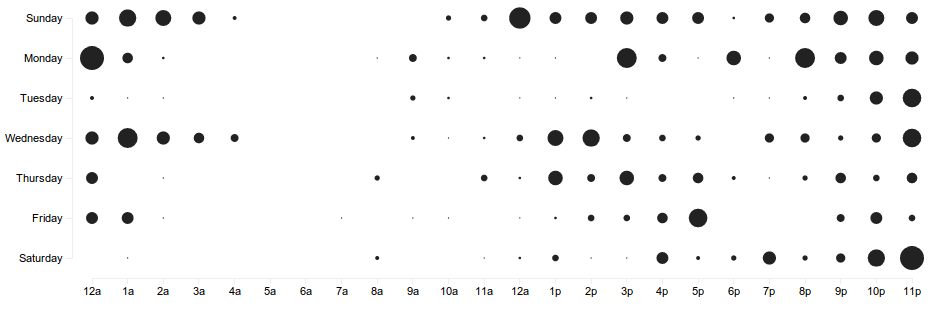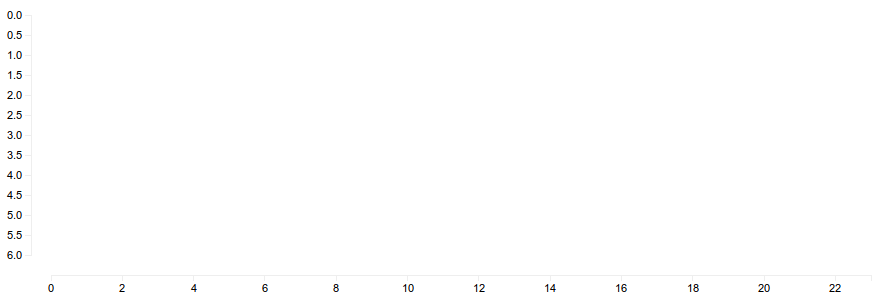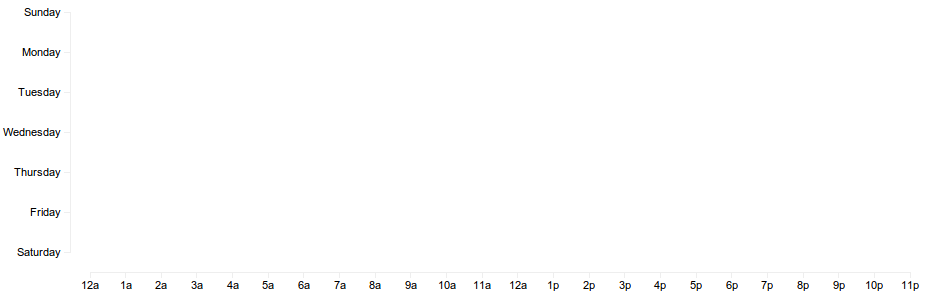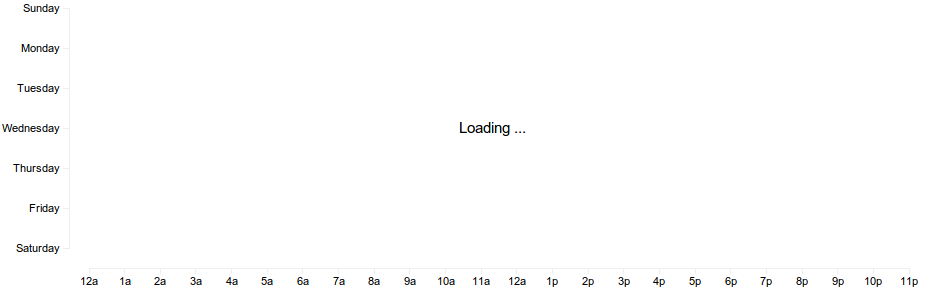swizec.com
👋 ready to invest in your career? Get the Senior Engineer Mindset book 20% off this week only

#### Senior Mindset Book

Get promoted, earn a bigger salary, work for top companies

# Quick scatterplot tutorial for d3.jsOne of the many interesting things Github does are punchcards for repositories that can tell you when people work on their code. Unfortunately, they're only per-repository and I was interested in per-user Github punchcards.

Collecting the data was fairly straightforward, finding a simple tutorial/example of a scatterplot in d3.js proved to be less than trivial.

## Scatterplotting

Drawing a scatterplot is nothing more than distributing data into buckets in a two dimensional space, then drawing a circle based on how many entities ended up in a particular bucket. Adding some colour gives us an extra dimension.

For starters, we're going to need some simple HTML and a bit of CSS to make things prettier.

```<style>  .axis path,  .axis line {    fill: none;    stroke: #eee;    shape-rendering: crispEdges;  }.css-13aqjzy{display:inline-block;}
.axis text {    font-family: sans-serif;    font-size: 11px;  }
.circle {    fill: #222;  }</style>
<div id="punchcard"></div>
<script src="https://d3js.org/d3.v2.min.js"></script><script src="script.js"></script>```

The div is where our scatterplot will end up. It doesn't need any styling since we'll do that with d3 directly, but splashing some CSS on stuff in the graph is going to make things look much better.

Going into script.js we start off by defining the width, height and padding for our graph. Due to d3's magic we'll later be able to change these and have the graph scale itself properly without further intervention.

`var w = 940,  h = 300,  pad = 20,  left_pad = 100,  Data_url = "/data.json";`

Next we define our scatterplot

`var svg = d3  .select("#punchcard")  .append("svg")  .attr("width", w)  .attr("height", h);`

This tells d3 that we want to put some svg in the punchcard div and how big we want it.

The next step is defining our x _and _y scales. They're helpful for translating data into x,y positions on the graph since they're usually not the same.

`var x = d3.scale    .linear()    .domain([0, 23])    .range([left_pad, w - pad]),  y = d3.scale    .linear()    .domain([0, 6])    .range([pad, h - pad * 2]);`

We specified that x _coordinates map from values between 0 and 23 to coordinates between the left padding and however big the graph is. Similarly for _y coordinates.

D3 will handle everything else for us.

Next we should define our axes since graphs without labeled axes are rather useless.

`var xAxis = d3.svg.axis().scale(x).orient("bottom"),  yAxis = d3.svg.axis().scale(y).orient("left");`

Essentially we've told the axes to use their corresponding scales and where we want the labels to end up.

Now it's finally time to draw something!

```svg  .append("g")  .attr("class", "axis")  .attr("transform", "translate(0, " + (h - pad) + ")")  .call(xAxis);
svg  .append("g")  .attr("class", "axis")  .attr("transform", "translate(" + (left_pad - pad) + ", 0)")  .call(yAxis);```As you can see, d3 was nice enough to figure out on its own how many ticks to draw and where to put them. This usually makes graphs more readable, but in our case we do want all the ticks.

All it takes is telling the axes how many ticks we want and while we're at it let's improve the labels as well.

`var xAxis = d3.svg    .axis()    .scale(x)    .orient("bottom")    .ticks(24)    .tickFormat(function (d, i) {      var m = d > 12 ? "p" : "a";      return d % 12 == 0 ? 12 + m : (d % 12) + m;    }),  yAxis = d3.svg    .axis()    .scale(y)    .orient("left")    .ticks(7)    .tickFormat(function (d, i) {      return [        "Sunday",        "Monday",        "Tuesday",        "Wednesday",        "Thursday",        "Friday",        "Saturday",      ][d];    });`

The .tickFormat method allows us to give d3 a function that calculates what a label should look like and we used .ticks to specify how many ticks we want.Much better, but since we'll be loading the data asynchronously, let's tell the user what's going on by placing "Loading ..." roughly in the middle of the plot.

`svg  .append("text")  .attr("class", "loading")  .text("Loading ...")  .attr("x", function () {    return w / 2;  })  .attr("y", function () {    return h / 2 - 5;  });``d3.json(Data_url, function (punchcard_data) {`

Within that function we are now going to draw the actual scatterplot.

The data I loaded is organized into triplets [day, hour, N] where the combination of day and hour tells us where to draw a circle and N tells us how big it should be.

We should define another scale for the radius of the circles.

`var max_r = d3.max(    punchcard_data.map(function (d) {      return d;    })  ),  r = d3.scale    .linear()    .domain([      0,      d3.max(punchcard_data, function (d) {        return d;      }),    ])    .range([0, 12]);`

Everything from zero to max_r will be mapped to radiuses between 0 and 12 pixels.

To make d3 put some data on our graph we need to tell it to load up our data into the graph and give a transformation that results in circles.

```svg.selectAll(".loading").remove();
svg  .selectAll("circle")  .data(punchcard_data)  .enter()  .append("circle")  .attr("class", "circle")  .attr("cx", function (d) {    return x(d);  })  .attr("cy", function (d) {    return y(d);  })  .attr("r", function (d) {    return r(d);  });```

After removing the "Loading ..." _we gave d3 our data, then said that for each datum a _circle should be appended to the graph and given attributes cx, cy and r that determine where this circle will be displayed and how big it's going to be.

Notice we're using functions to compute these values, but because we're lazy we just rely on d3 doing all of the actual calculations with the x, y and r scales we defined earlier.On pictures everything looks great now, but the circles appear very suddenly. Wouldn't it be nice if there was a sexy transition going on?

To do that we shove a .transition() and a .delay(800) before defining the radius.

`svg  .selectAll("circle")  .data(punchcard_data)  .enter()  .append("circle")  .attr("class", "circle")  .attr("cx", function (d) {    return x(d);  })  .attr("cy", function (d) {    return y(d);  })  .transition()  .duration(800)  .attr("r", function (d) {    return r(d);  });`

Now the circles appear in a lovely 800 millisecond transition.

Here's the full javascript code:

```var w = 940,  h = 300,  pad = 20,  left_pad = 100,  Data_url = "/data.json";
var svg = d3  .select("#punchcard")  .append("svg")  .attr("width", w)  .attr("height", h);
var x = d3.scale    .linear()    .domain([0, 23])    .range([left_pad, w - pad]),  y = d3.scale    .linear()    .domain([0, 6])    .range([pad, h - pad * 2]);
var xAxis = d3.svg    .axis()    .scale(x)    .orient("bottom")    .ticks(24)    .tickFormat(function (d, i) {      var m = d > 12 ? "p" : "a";      return d % 12 == 0 ? 12 + m : (d % 12) + m;    }),  yAxis = d3.svg    .axis()    .scale(y)    .orient("left")    .ticks(7)    .tickFormat(function (d, i) {      return [        "Sunday",        "Monday",        "Tuesday",        "Wednesday",        "Thursday",        "Friday",        "Saturday",      ][d];    });
svg  .append("g")  .attr("class", "axis")  .attr("transform", "translate(0, " + (h - pad) + ")")  .call(xAxis);
svg  .append("g")  .attr("class", "axis")  .attr("transform", "translate(" + (left_pad - pad) + ", 0)")  .call(yAxis);
svg  .append("text")  .attr("class", "loading")  .text("Loading ...")  .attr("x", function () {    return w / 2;  })  .attr("y", function () {    return h / 2 - 5;  });
d3.json(Data_url, function (punchcard_data) {  var max_r = d3.max(      punchcard_data.map(function (d) {        return d;      })    ),    r = d3.scale      .linear()      .domain([        0,        d3.max(punchcard_data, function (d) {          return d;        }),      ])      .range([0, 12]);